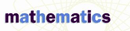Related Topics

# Quadratic Formula and Equations - Research Article from Macmillan Science Library: Mathematics

This encyclopedia article consists of approximately 5 pages of information about Quadratic Formula and Equations.
 This section contains 1,466 words (approx. 5 pages at 300 words per page) View a FREE sampleA quadratic equation is an equation of the second degree, meaning that for an equation in x, the greatest exponent on x is 2. Quadratics most commonly refer to vertically oriented parabolas—that is, parabolas that open upward or downward. The graph of a vertically oriented parabola has the shape of a rounded "v," and the bottom-most (or top-most) point is called the vertex.

The equation for a parabola is usually written in either standard or vertex form; however, the standard form is more commonly used to solve for the x-intercepts, or roots. The standard form is y = ax2+ bx + c for any real numbers a, b, c where a ≠ 0. The vertex form is y - k = a(x - b)2 with vertex (b, k) and where a ≠ 0.

Because x-intercepts are the points at which the graph crosses the x-axis...

(read more)

 This section contains 1,466 words (approx. 5 pages at 300 words per page) View a FREE sampleMore summaries and resources for teaching or studying Quadratic Formula and Equations.
CopyrightsQuadratic Formula and Equations from Macmillan. Copyright © 2001-2006 by Macmillan Reference USA, an imprint of the Gale Group. All rights reserved.